• 虽然有一些博文已经介绍了一些方法，但是我在调研和绘制时发现，大部分的博文中都用了复杂的for循环语句来实现（可能是因为我太笨，看不懂for循环），并且大部分都不是针对DataFrame来做累计概率分布的，都是做简单...

累积分布函数(Cumulative Distribution Function)，又叫分布函数，是概率密度函数的积分，能完整描述一个实随机变量X的概率分布。在电子器件制造中常常用于描述器件失效分布，可以明显的表示出在特定数值下的器件概率分布占比；
虽然有一些博文已经介绍了一些方法，但是我在调研和绘制时发现，大部分的博文中都用了复杂的for循环语句来实现（可能是因为我太笨，看不懂for循环），并且大部分都不是针对DataFrame来做累计概率分布的，都是做简单列表，在解决问题的过程中，我发现了自己认为更加简单，易用的代码实现方法，在这里与大家分享，希望对大家有所帮助；
首先，介绍解决问题的思路
众所周知，累计概率分布就是对于可选变量由小到大排列后，各个变量的累计概率形成的，因此：

**1）将所要处理的数据的所有可能取值中的唯一值以及唯一值出现的频数得出来；

2）所有数据的总数我们需要知道，因为每个数据出现频数除以数据总数才能获得该数据的概率；

3）将数据列表从小到大排列，然后将每个数据出现的概率进行叠加，这里需要用到cumsum函数；

4）绘制累计分布图**

然后，就是具体的代码分组讲解：
1)将所要处理的数据的所有可能取值中的唯一值以及唯一值出现的频数得出来；

import pandas as pd
import matplotlib.pyplot as plt
import numpy as np

这里是需要用到的模块，在程序最开始先调用，就像做菜准备好调料一样；

#读取待处理数据

以下是运行代码的终端显示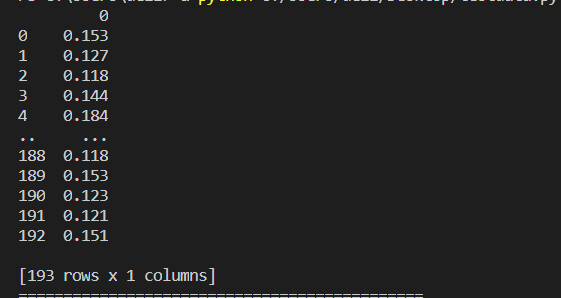数据类型为只有一列的dataframe格式；

#获取数据的总数
denominator=len(data)
#将数据转换为Series
Data=pd.Series(data)
#利用value_counts方法进行分组频数计算
Fre=Data.value_counts()
#对获得的表格整体按照索引自小到大进行排序
Fre_sort=Fre.sort_index(axis=0,ascending=True)

得到的Fre_sort数据如下所示：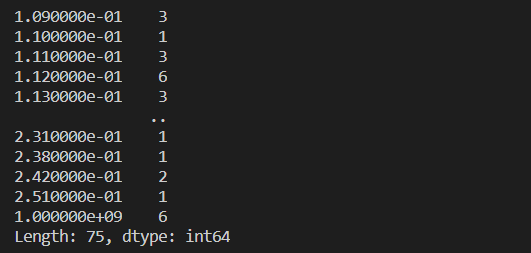2）所有数据的总数我们需要知道，因为每个数据出现频数除以数据总数才能获得该数据的概率；

#重置表格索引
Fre_df=Fre_sort.reset_index()
#将频数转换成概率
Fre_df=Fre_df/denominator
#将列表列索引重命名
Fre_df.columns=['Rds','Fre']

得到的列表输出如下所示：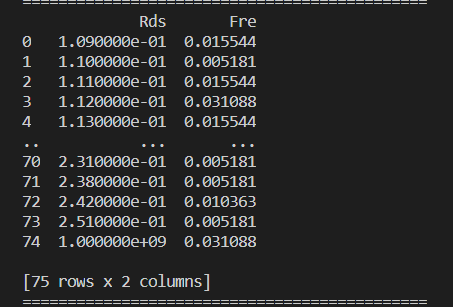3）将数据列表从小到大排列，然后将每个数据出现的概率进行叠加，这里需要用到cumsum函数；

#利用cumsum函数进行概率的累加并按照顺序添加到表格中
Fre_df['cumsum']=np.cumsum(Fre_df['Fre'])

数据表格输出如下所示：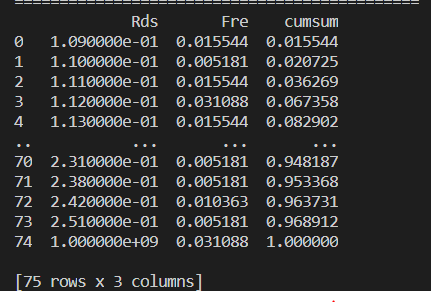#创建画布
plot=plt.figure()
#只有一张图，也可以多张
#按照Rds列为横坐标，累计概率分布为纵坐标作图
ax1.plot(Fre_df['Rds'],Fre_df['cumsum'])
#图的标题
ax1.set_title("CDF")
#横轴名
ax1.set_xlabel("Rds")
#纵轴名
ax1.set_ylabel("P")
#横轴的界限
ax1.set_xlim(0.1,0.5)
#图片显示
plt.show()

4)绘制累计分布图
图片输出如下所示：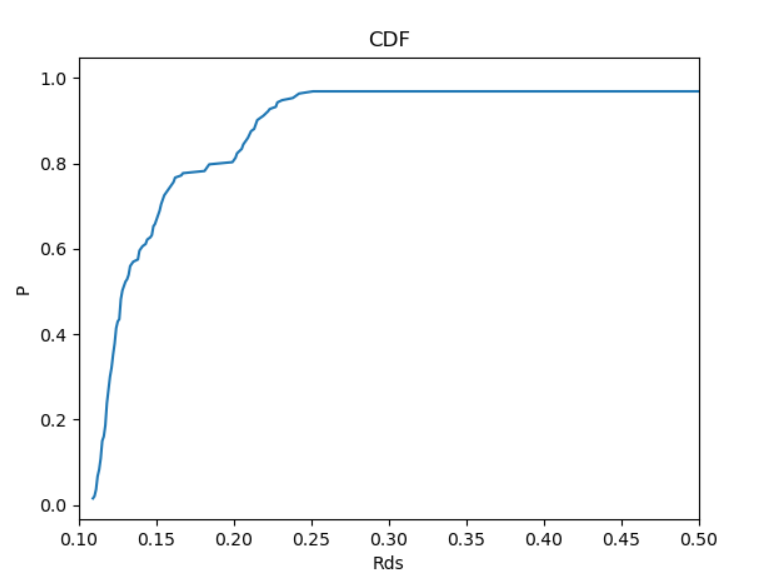完整代码如下所示：

import pandas as pd
import matplotlib.pyplot as plt
import numpy as np
data=[]
denominator=len(data)#分母数量
Data=pd.Series(data)#将数据转换为Series利用分组频数计算
Fre=Data.value_counts()
Fre_sort=Fre.sort_index(axis=0,ascending=True)
Fre_df=Fre_sort.reset_index()#将Series数据转换为DataFrame
Fre_df=Fre_df/denominator#转换成概率
Fre_df.columns=['Rds','Fre']
Fre_df['cumsum']=np.cumsum(Fre_df['Fre'])
plot=plt.figure()
ax1.plot(Fre_df['Rds'],Fre_df['cumsum'])
ax1.set_title("CDF")
ax1.set_xlabel("Rds")
ax1.set_ylabel("P")
ax1.set_xlim(0.1,0.5)
plt.show()

注意事项：

Data=pd.Series(data)#将数据转换为Series利用分组频数计算
Fre=Data.value_counts()

2）在对Fre数据表格进行排序时，需要用到Series和DataFrame的sort_index函数，其中axis=0代表按照行索引进行排序，默认是升序，降序用ascending=False来限定，如果按照特定行或列，可以在by参数中进行陷定；

Fre=Data.value_counts()
Fre_sort=Fre.sort_index(axis=0,ascending=True)

3）cumsum函数特别适合于进行CDF图绘制，与for循环相比，效率应该更高；

Fre_df['cumsum']=np.cumsum(Fre_df['Fre'])

4）图片的横坐标界限需要细化，否则，由于里面的个别异常点导致图像的可视化程度降低，也可以通过布尔索引，将异常值去掉；

展开全文python 数据分析 可视化
• Python绘制概率分布直方图

千次阅读 2021-12-13 12:46:25
Python绘制概率分布直方 文章目录Python绘制概率分布直方一、代码1、案例一2、案例二二、运行效果1、案例一2、案例二 一、代码 1、案例一 import random import numpy as np import matplotlib.pyplot as plt X...

Python绘制概率分布直方图

一、代码

1、案例一

import random
import numpy as np
import matplotlib.pyplot as plt

X = np.random.normal(loc=10, scale=2, size=(1, 1000))

Y = np.random.normal(loc=10, scale=1.0, size=(1, 1000))

P = np.ones(1000)
P[:600] = 0
np.random.shuffle(P)
# print(P)

Z = X + Y * P

plt.hist(Z, density=True, bins=150)
plt.show()

2、案例二

import random
import numpy as np
import matplotlib.pyplot as plt

n = 1000
# p = 0.4
U = []

for i in range(1000):

X = np.random.normal(loc=10, scale=2, size=(1, n))

Y = np.random.normal(loc=10, scale=1.0, size=(1, n))

P = np.ones(n)
P[:600] = 0
np.random.shuffle(P)

Z = X + Y * P

a = 1 / pow(n * np.var(Z), 1/2)

b = sum(Z) - n * np.mean(Z)

u = a * b

U.append(u)

plt.hist(U, density=True, bins=60)
plt.show()

二、运行效果

1、案例一2、案例二最后，感谢大家的阅读与支持，谢谢大家了啦。

展开全文python 深度学习 机器学习 概率直方图
• 如何利用R语言画出含有置信区间的累积概率分布图 数据导入 1.data<c(0.327,0.048,0.436,0.501,0.289,0.050,0.099,0.010,0.135,0.000) 或data<-scan(‘data.text’) 2.install.packages(“sROC”) 3.library...

如何利用R语言画出含有置信区间的累积概率分布图

数据导入
1.data<c(0.327,0.048,0.436,0.501,0.289,0.050,0.099,0.010,0.135,0.000)
或data<-scan(‘data.text’)
2.install.packages(“sROC”)
3.library(sROC)
4.plot(kCDF( a=kCDF(x=data,bw=0.06757,kernel=(‘normal’),from=0,to=1))#默认置信区间为0.95
5.plot(kCDF( a=kCDF(x=data,bw=0.06757,kernel=(‘normal’),from=0,to=1),alpha=0.2)#置信区间改为0.8
6.result<-kCDF( a=kCDF(x=data,bw=0.06757,kernel=(‘normal’),from=0,to=1)#将累积概率分布图导入result
7.x<-result
8.Fha<-result
9.f<-data.frame(s,Fhat)
10.write.table(f,file=“f.csv”),sep=",",row.names = FALSE)

展开全文r语言

这里有一个使用pymc的方法。该方法在混合模型中使用固定数量的组分(n_分量)。您可以尝试附加一个prior-to-n-u组件并在该previor上采样。或者，您可以选择一些合理的东西，或者从我的其他答案中使用网格搜索技术来估计组件的数量。在下面的代码中，我使用了10000次迭代，但这可能不足以获得良好的结果。我建议使用大得多的磨合机。我也有点武断地选择了优先权，所以这些可能是值得一看的东西。你得试试看。祝你好运。这是密码。在import numpy as np

import pymc as mc

import scipy.stats as stats

from matplotlib import pyplot

def generate_random_histogram():

# Random bin locations between 0 and 100

bin_locations = np.random.rand(10,) * 100

bin_locations.sort()

# Random counts on those locations

bin_counts = np.random.randint(50, size=len(bin_locations))

return {'loc': bin_locations, 'count':bin_counts}

def bin_widths(loc):

widths = []

for i in range(len(loc)-1):

widths.append(loc[i+1] - loc[i])

widths.append(widths[-1])

widths = np.array(widths)

return widths

def mixer(name, weights, value=None):

n = value.shape

def logp(value, weights):

vals = np.zeros(shape=(n, weights.shape), dtype=int)

vals[:, value.astype(int)] = 1

return mc.multinomial_like(x = vals, n=1, p=weights)

def random(weights):

return np.argmax(np.random.multinomial(n=1, pvals=weights[0,:], size=n), 1)

result = mc.Stochastic(logp = logp,

doc = 'A kernel smoothing density node.',

name = name,

parents = {'weights': weights},

random = random,

trace = True,

value = value,

dtype = int,

observed = False,

cache_depth = 2,

plot = False,

verbose = 0)

return result

def create_model(lowers, uppers, count, n_components):

n = np.sum(count)

lower = min(lowers)

upper = min(uppers)

locations = mc.Uniform(name='locations', lower=lower, upper=upper, value=np.random.uniform(lower, upper, size=n_components), observed=False)

precisions = mc.Gamma(name='precisions', alpha=1, beta=1, value=.001*np.ones(n_components), observed=False)

weights = mc.CompletedDirichlet('weights', mc.Dirichlet(name='weights_ind', theta=np.ones(n_components)))

choice = mixer('choice', weights, value=np.ones(n).astype(int))

@mc.observed

def histogram_data(value=count, locations=locations, precisions=precisions, weights=weights):

if hasattr(weights, 'value'):

weights = weights.value

lower_cdfs = sum([weights[0,i]*stats.norm.cdf(lowers, loc=locations[i], scale=np.sqrt(1.0/precisions[i])) for i in range(len(weights))])

upper_cdfs = sum([weights[0,i]*stats.norm.cdf(uppers, loc=locations[i], scale=np.sqrt(1.0/precisions[i])) for i in range(len(weights))])

bin_probs = upper_cdfs - lower_cdfs

bin_probs = np.array(list(upper_cdfs - lower_cdfs) + [1.0 - np.sum(bin_probs)])

n = np.sum(count)

return mc.multinomial_like(x=np.array(list(count) + ), n=n, p=bin_probs)

@mc.deterministic

def location(locations=locations, choice=choice):

return locations[choice.astype(int)]

@mc.deterministic

def dispersion(precisions=precisions, choice=choice):

return precisions[choice.astype(int)]

data_generator = mc.Normal('data', mu=location, tau=dispersion)

return locals()

# Generate the histogram

hist = generate_random_histogram()

loc = hist['loc']

count = hist['count']

widths = bin_widths(hist['loc'])

lowers = loc - widths

uppers = loc + widths

# Create the model

model = create_model(lowers, uppers, count, 5)

# Initialize to the MAP estimate

model = mc.MAP(model)

model.fit(method ='fmin')

# Now sample with MCMC

model = mc.MCMC(model)

model.sample(iter=10000, burn=9000, thin=300)

# Plot the mu and tau traces

mc.Matplot.plot(model.trace('locations'))

pyplot.show()

# Get the samples from the fitted pdf

sample = np.ravel(model.trace('data')[:])

# Plot the original histogram, sampled histogram, and pdf

lower = min(lowers)

upper = min(uppers)

kde = stats.gaussian_kde(sample)

x = np.arange(0,100,.1)

y = kde(x)

fig = pyplot.figure()

pyplot.xlim(lower,upper)

ax1.bar(loc, count, width=widths)

ax2.hist(sample, bins=loc)

ax3.plot(x, y)

pyplot.show()展开全文• 写作业需要画一个二维概率分布函数的图像，便想到可以利用matlab来画，但还没有找到很简洁的方法，用surf大概画了下matlab
• 一、常见的概率分布表1.1　概率分布分类表连续随机变量分布连续统计量分布离散随机变量分布分布分布二项分布连续均匀分布非中心 分布离散均匀分布(Gamma)分布分布几何分布指数分布非中心 分布超几何分布正态分布分布...
• 我不太清楚你想要什么，所以我猜，...(使用上面显示的标记绘制，而不是更传统的阶梯…)import scipy.statsimport numpy as npimport matplotlib.pyplot as plt# 100 values from a normal distribution with a s...
• 绘制概率密度

千次阅读 2021-02-04 07:12:24
最常见的方法是使用fill_between对置信区间之间的区域进行着色。例如：import numpy as npnp.random.seed(1977)import matplotlib.pyplot as plt# Generate data...x_obs = np.linspace(-2, 2, 20)true_model = [0.2...
• 本文介绍了如何读取H5文件模型的权重，然后用MATLAB中绘制权重的概率密度图和分布图python 人工智能 github
• 今天使用python画了几个好玩的3D展示，现在分享给大家。先贴上图片使用的python工具包为：from matplotlib import pyplot as pltimport numpy as npfrom mpl_toolkits.mplot3d import Axes3D在贴代码之前，有必要...
• 数据来自混合高斯分布![](http://www.forkosh.com/mathtex.cgi? \large Y=0.3X_1+0.6X_2+0.1X_3)随机变量的均值如下, 各分量之间相互独立, 方差100mu1=[-55,0,90];s1=100*eye(3,3);mu2=[-30,-10,70];s2=s1;mu3=[-20,...
• 赛马正态分布图1.制作IQ数据图import numpy as npimport pandas as pdimport matplotlib.pyplot as plt#使用%matplotlib命令可以将matplotlib的图表直接嵌入到Notebook之中，或者使用指定的界面库显示图表，它有一个...
•   那么，本文就将用seaborn来实现联合分布图绘制。seaborn是一个基于matplotlib的Python数据可视化模块，借助于其，我们可以通过较为简单的操作，绘制出各类动人的图片。   首先，引入需要的模块。 import ...python seaborn pairplot 联合分布图
• 我给出了P(x1 … n)离散独立概率值,它代表了例如发生X的可能性.我想要一个问题的通用代码：X发生的概率是在同一时间发生的0-n次？例如：给定：每辆车(A,B,C)停放的3个概率P(A),P(B),P(C).问题是：哪个概率不会有车,...
• 简单对概率分布函数进行一个描述，在概率论中要研究一个随机变量ξ取值小于某一数值x的概率，这概率是x的函数，称这种函数为随机变量ξ的分布函数。 F(x)=P(X<x),F(+∞)=1,F(−∞)=0F(x)=P(X<x),F(+\infin)=1,...
• 本文演示了如何通过python绘制双y轴、箱线图、概率分布三种图形，并给出了具体案例源代码供读者参考。 绘制双y轴图形 效果如下 代码如下 import numpy as np import matplotlib.pyplot as plt..python
• 使用C++分析RGB和YUV格式图像的三个通道的概率分布及其熵一、使用C++计算熵的方法二、使用C++分析RGB格式的图像1、读取图像并分离RGB三通道2、计算RGB三通道的概率分布3、计算RGB三通道的熵4、输出RGB分量概率分布及...
• >> x=0:6.5:650;y=0:27:2700;[x,y]=meshgrid(x,y);F=(669126134152931648175758856558983806728427764752864631.*35184372088832.^(1819./5000).*3695207012694701.^(3181./5000).*gamma(3181./5000))./642775...
• 连续变量的概率分布可以通过绘制概率密度函数( PDF )来可视化。一个结果的概率密度表示随机变量取该值的相对可能性。它可以取任何正值并且积分总是1。1描述了一个离散分布列（直方表示）与概率密度函数函数的...计算机视觉 全概率公式 贝叶斯定理 联合概率 边缘概率
• EXCEL 正态分布公式你是要取在33到38.5出现的概率吧用正态分布概率密度函数相减即可即=NORMDIST(38.5,36,2,TRUE) - NORMDIST(33,36,2,TRUE)Excel里面如何做正态分布图一、直方图将数据输入到EXCEL同一(这里放入A列)...
• stata如何画分布图

千次阅读 2021-01-12 23:05:35
菜单操作 Plots 选择横、纵坐标所代表的变量 Titles 标题 Title( )......LOGO Stata中的图形制作 主要内容: 1、图形制作的基本命令与相关操作 2、直方、散点绘制 3、条形、饼图的绘制 4、图形的保存、合并与...
• seid=16338053070486680597&...%%绘制直方+概率密度函数+累积分布函数 %矩阵A是random生成0-1的随机数，或读入自己的数据 A = rand(1,500)*50; %求A的最大、最小、均值 maxA = max(A); minA = min(A); meanA = meanmatlab 开发语言 统计模型
• python绘制累积正态分布概率图 累积正态分布概率图，即正态分布函数的积分形式。其公式为： 去掉积分符号就是正态分布的公式。 以下是实现的代码。 // A code block import numpy as np import matplotlib.pyplot ...python 数据分析
• 样本概率图绘制 二、语法 1.p = capaplot（data，specs） 估计输入矢量数据中观测值的均值和方差，并绘制所得T分布的pdf。 数据中的观察值假定为正态分布。 输出p是来自估计分布的新观测值将落在由两个元素组成...matlab 概率图
• Python绘制的二项分布概率图示例发布时间：2020-09-08 00:10:14来源：脚本之家阅读：92作者：hellBaron本文实例讲述了Python绘制的二项分布概率图。分享给大家供大家参考，具体如下：问题：抛硬币，20次，每一次朝上...
• 本例中原始数据：51.7,50.6,57.9,56.9,56.7,56.7,55.3,56.1,53.7,54.5,56.9,51.9,52.1,55.1,54.9,54.7,55.3,55.3,54.5,54.9,54.5,55.3,54.9,54.3,53.7,53.5,53.7,53.1,54.5,53.1,53.9,53.5,53.3,53.9,53.5,53.5,52.5...
• 用excel、matlab、python绘制正态分布图excel绘图matlab绘图python绘图 为加深对统计知识的理解，老师要求用excel和matlab绘图，在这想介绍3个常见的绘图软件来绘制正态分布密度函数曲线和正态分布分布函数曲线。 ...matlab python
• 本文档通过MATLAB来绘制二维随机变量(X,Y)的联合概率密度函数。其中X服从标准正态分布，Y服从均匀分布。 【例题】已知随机变量X与Y相互独立，X~N(0,1);Y在区间[0,2]上服从均匀分布。求： （1）二维随机变量(X,Y)的...matlab...